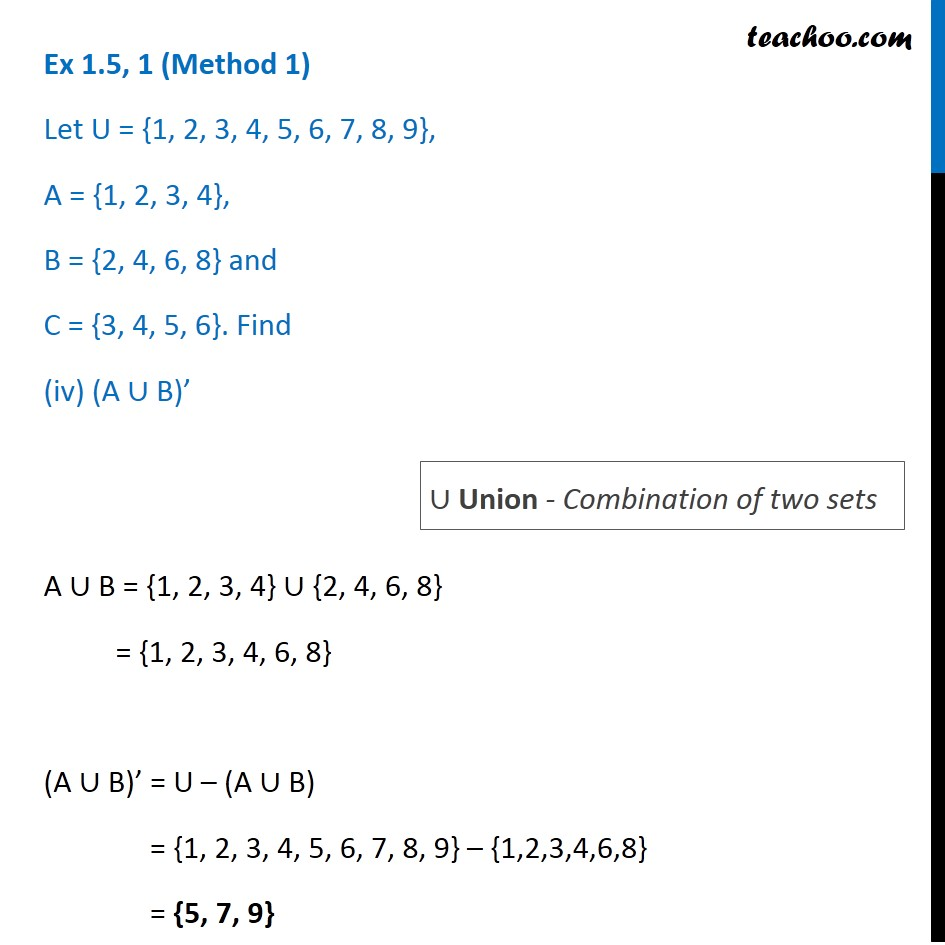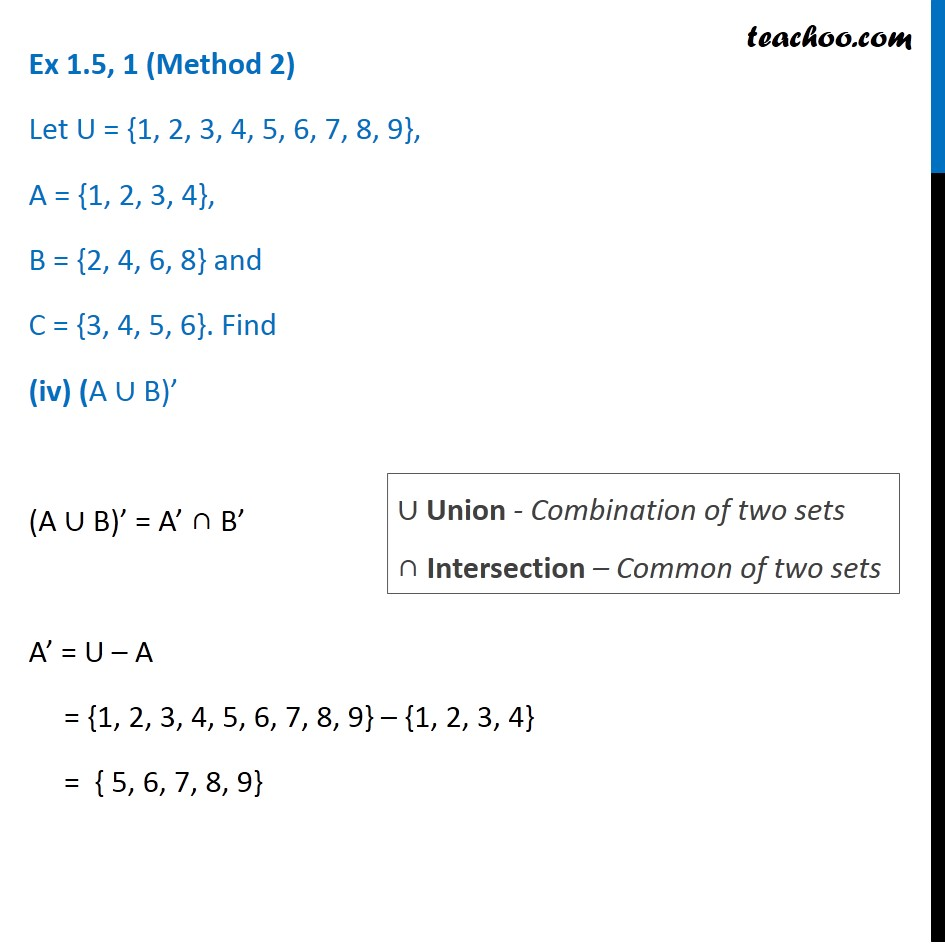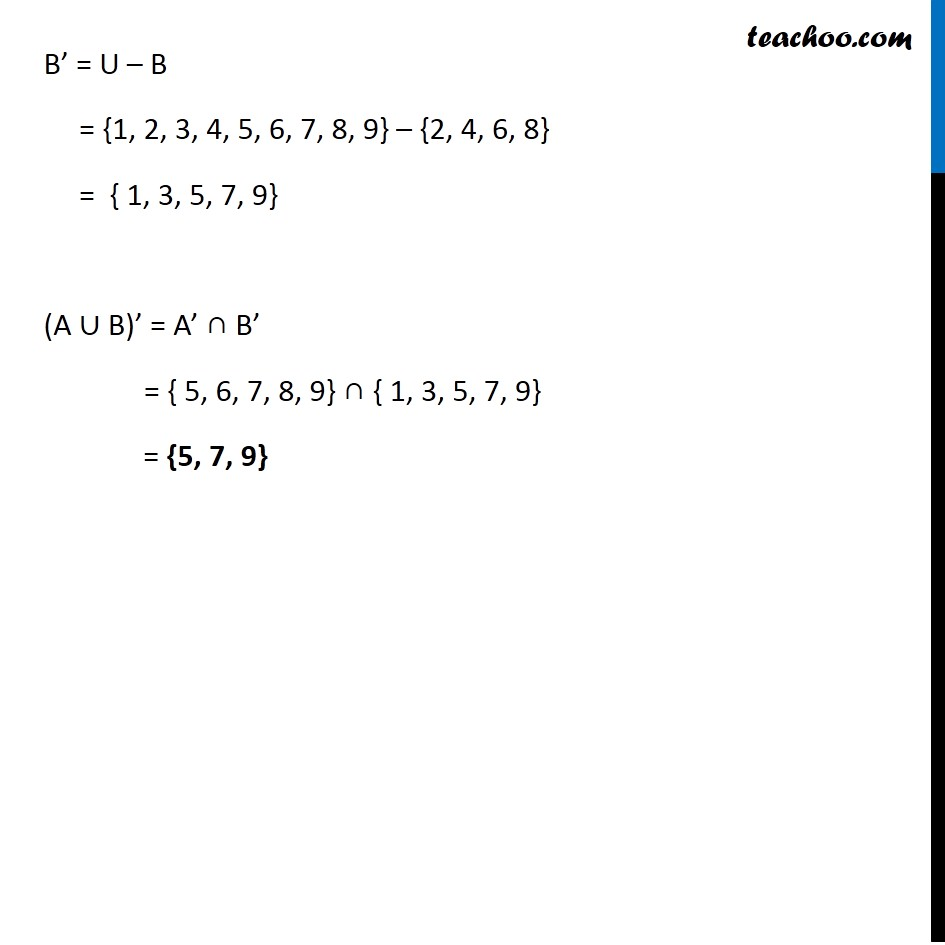1. Chapter 1 Class 11 Sets (Term 1)
2. Serial order wise
3. Ex 1.5

Transcript

Ex 1.5, 1 (Method 1) Let U = {1, 2, 3, 4, 5, 6, 7, 8, 9}, A = {1, 2, 3, 4}, B = {2, 4, 6, 8} and C = {3, 4, 5, 6}. Find (A ∪ B)’ A ∪ B = {1, 2, 3, 4} ∪ {2, 4, 6, 8} = {1, 2, 3, 4, 6, 8} (A ∪ B)’ = U – (A ∪ B) = {1, 2, 3, 4, 5, 6, 7, 8, 9} – {1,2,3,4,6,8} = {5, 7, 9} ∪ Union - Combination of two sets Ex 1.5, 1 (Method 2) Let U = {1, 2, 3, 4, 5, 6, 7, 8, 9}, A = {1, 2, 3, 4}, B = {2, 4, 6, 8} and C = {3, 4, 5, 6}. Find (iv) (A ∪ B)’ (A ∪ B)’ = A’ ∩ B’ A’ = U – A = {1, 2, 3, 4, 5, 6, 7, 8, 9} – {1, 2, 3, 4} = { 5, 6, 7, 8, 9} ∪ Union - Combination of two sets ∩ Intersection – Common of two sets B’ = U – B = {1, 2, 3, 4, 5, 6, 7, 8, 9} – {2, 4, 6, 8} = { 1, 3, 5, 7, 9} (A ∪ B)’ = A’ ∩ B’ = { 5, 6, 7, 8, 9} ∩ { 1, 3, 5, 7, 9} = {5, 7, 9}